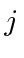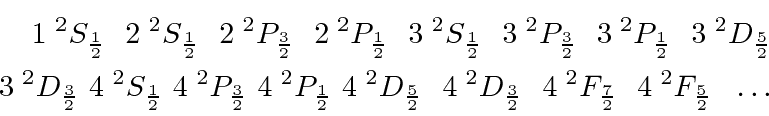## Spectroscopic Notation

A common way to name states in atomic physics is to use spectroscopic notation. It is essentially a standard way to write down the angular momementum quantum numbers of a state. The general form is, whereis the principal quantum number and will often be omitted,is the total spin quantum number (is the number of spin states),refers to the orbital angular momentum quantum numberbut is written asfor, andis the total angular momentum quantum number.

A quick example is the single electron states, as we find in Hydrogen. These are:All of these have the pre-superscript 2 because they are all spin one-half. There are twovalues for each.

For atoms with more than one electron, the total spin state has more possibilities and perhaps several ways to make a state with the same quantum numbers.

Jim Branson 2013-04-22